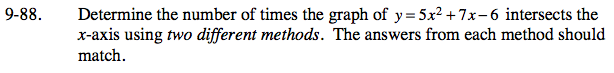### Home > CCA > Chapter 9 > Lesson 9.3.2 > Problem9-88

9-88.

Determine the number of times the graph of y = 5x2 + 7x − 6 intersects the x‑axis using two different methods. The answers from each method should match. Homework Help ✎Possible methods include:
- Make a table
- Factor and use the Zero Product Property
- Use the Quadratic Formula
- Rewrite the equation in graphing form by completing the square